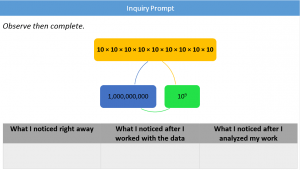# Exponents, Just the BeginningThe exponent of a number shows how many times to multiply it; It is repeated multiplication. In Grade 5, students are introduced to exponents while writing big numbers in expanded form. At this level, they are required to use whole number exponents to denote powers of 10, use the new terminology (base, power, squared, and cubed) and practice moving between exponential notation, expanded notation, and standard notation.

This learning experience is part of the unit plan titled “Numbers” under the central idea “Numbers can be written and represented in different forms.”

The Inquiry Prompt
Prompts are mathematical statements that promote students’ curiosity, develop mathematical knowledge, challenge them to discover new concepts, and provide opportunities for different forms of thinking, including induction and deduction approaches. (As defined by Andrew Blair, @inquirymathshttp://www.inquirymaths.com/)

Learners were divided into groups and each group took an inquiry prompt similar to the following. All the prompts were about the power of 10, with different power for each group. Learners were asked to write what they noticed at three different stages.Learners demonstrated a deep inquiry process, connecting their prior knowledge to develop their understanding of the mathematical structure of the prompt. They interpreted the three cards of the prompts and wondered if they represent the same value. The learners were able to conclude that the yellow and the blue cards are equal. However, a common mistake came to some learner’s mind when finding the product of the yellow card. They wrote 100 × 100 equals to 200! Teachers were ready with their scaffolding questions so they asked learners to reflect and go back to the definition of multiplication as repeated addition and to how we multiply by the multiples of 10. Therefore, learners connected the yellow card to the blue one and started wondering about the meaning and the value of the green one. The following are samples of the leaners’ thoughts about the prompt.At the final stage, learners drew the following conclusion and asked the following questions:

Conclusions

• The “small number” (power) represents the number of zero in the number written in word form.
• All the cards are equal, but they are written in different ways.
• Repeated multiplication is written in different forms.

Questions

• What do we call repeated multiplication?
• What is the value of 100?
• If 1,000,000,000 is equal to , so is 9,000,000,000 equal to ?
• Why do we use exponents?
• Can we do calculation (add, subtract, multiply, or divide) with exponents?
• Do we use power only with base 10? Could it be applied to other numbers?
• Is an exponent written only in standard form?
• Why do we learn about exponents and where can we apply them in real life?

Teachers updated their planning based on the leaners’ wonderings and proceeded with the cycle of inquiry until the learners reached the understanding of the required generalizations:

• Exponents are used to represent repeated multiplication.
• Exponential notation simplifies the writing of numbers in expanded form.

The Assessment

In order to assess the learners’ understanding, teachers provided them with the following WODB activity where learners apply their mathematical and reasoning skills to decide which of the four items does not belong and then justify why their choice is valid.The following are samples of the leaners’ answers.Fifth grade teachers (Noor Taweel @TawilNoor, Farah Darazi @DaraziFarah, Nadine Assaf  @Nadine_assaff, Nancy Karout @KaroutNancyk, Zeina Zaatary @ZeinaZaatary, Sasha Ghosn @sashgh, Rasha Hamzawi @racha_hamzawi) collaborated very well to implement this engagement in their classroom.